maths > calculus-limits

Understanding Limits : Examples

what you'll learn...

Limits by Numerator and Denominator

Slopes of the numerator and denominator at the point x=a$x = a$ decide the limits of f(x)$f \left(x\right)$ at x=a$x = a$.
»  eg: f(x)=2x4x2$f \left(x\right) = \frac{\textcolor{\mathrm{de} e p s k y b l u e}{2 x - 4}}{\textcolor{c \mathmr{and} a l}{x - 2}}$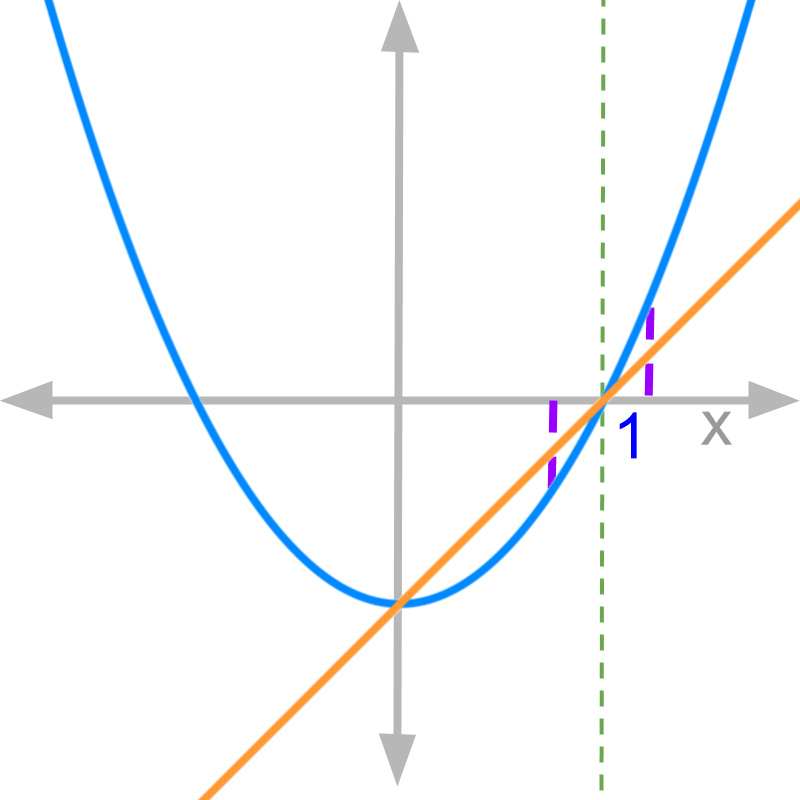→  slope of numerator at x=2$x = 2$ is 2$2$

→  slope of denominator at x=2$x = 2$ is $1$

→  Both LHL and RHL limits $= \frac{2}{1} = 2$

linear slopes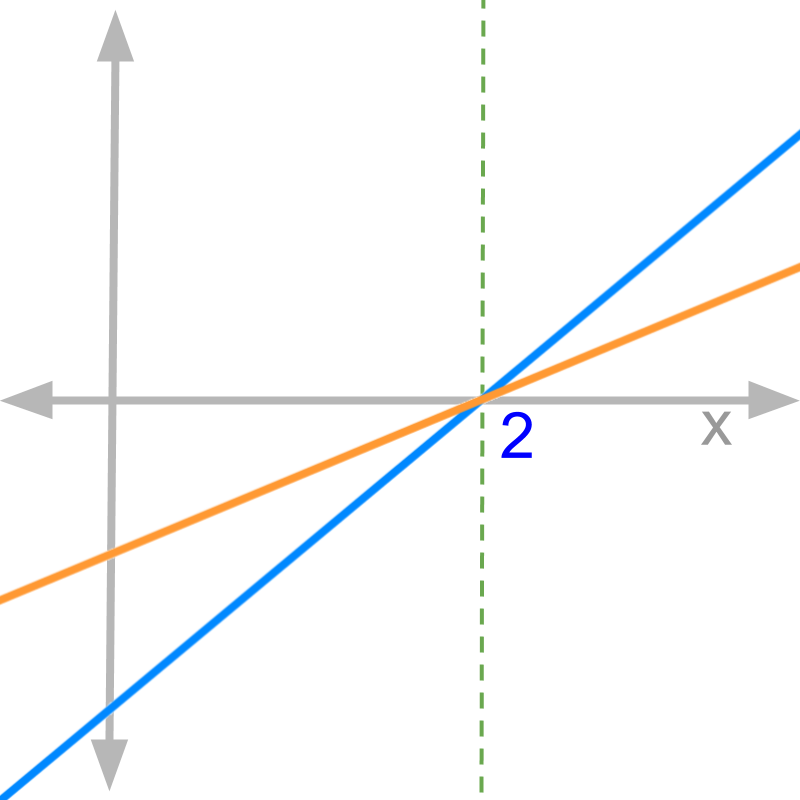Consider the graphs of numerator (in blue) and denominator (in orange) of function $f \left(x\right)$.

The value of ${\lim}_{x \to 2} f \left(x\right) > 1$. This is because, the slope of numerator is greater than the denominator.

The function given is $f \left(x\right) = \frac{\textcolor{\mathrm{de} e p s k y b l u e}{2 x - 4}}{\textcolor{c \mathmr{and} a l}{x - 2}}$. The slope of the numerator is $2$ and slope of the denominator is $1$.

Left-hand-limit $x = 2 - \delta$ of
$\frac{\textcolor{\mathrm{de} e p s k y b l u e}{2 x - 4}}{\textcolor{c \mathmr{and} a l}{x - 2}}$
$\quad \quad = \frac{\textcolor{\mathrm{de} e p s k y b l u e}{2 \left(2 - \delta\right) - 4}}{\textcolor{c \mathmr{and} a l}{2 - \delta - 2}}$
$\quad \quad = \frac{\textcolor{\mathrm{de} e p s k y b l u e}{4 - 2 \delta - 4}}{\textcolor{c \mathmr{and} a l}{- \delta}}$
$\quad \quad = \frac{\textcolor{\mathrm{de} e p s k y b l u e}{- 2 \delta}}{\textcolor{c \mathmr{and} a l}{- \delta}}$
$\quad \quad = 2$

Similarly, the right-hand-limit can be worked out to $2$.

negative slope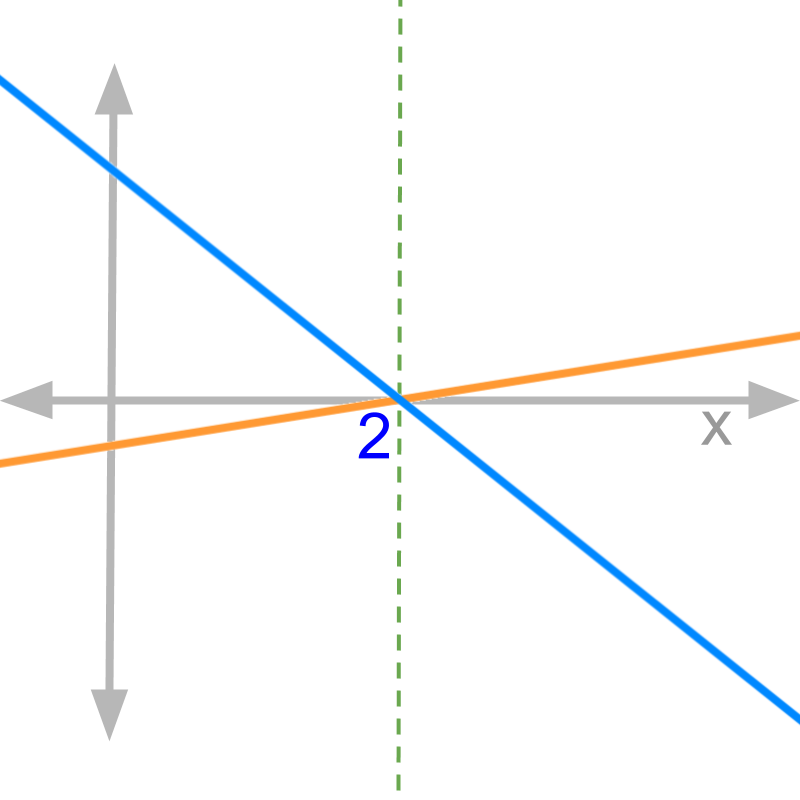Consider the graphs of numerator (in blue) and denominator (in orange) of function $f \left(x\right)$.

The value of ${\lim}_{x \to 2} f \left(x\right) < - 1$. This is because, the slope of the numerator is negative and decreasing steeper than the denominator.

The function given is $f \left(x\right) = \frac{\textcolor{\mathrm{de} e p s k y b l u e}{6 - 3 x}}{\textcolor{c \mathmr{and} a l}{.5 x - 1}}$. The slope of the numerator is $- 3$ and the slope of the denominator is $0.5$.

Right-hand-limit $x = 2 + \delta$
$\frac{\textcolor{\mathrm{de} e p s k y b l u e}{6 - 3 \left(2 + \delta\right)}}{\textcolor{c \mathmr{and} a l}{0.5 \left(2 + \delta\right) - 1}}$
$\quad \quad = \frac{\textcolor{\mathrm{de} e p s k y b l u e}{- 3 \delta}}{\textcolor{c \mathmr{and} a l}{0.5 \delta}}$
$\quad \quad = - 6$

Similarly, left hand limit can be worked out as $- 6$.

same slopes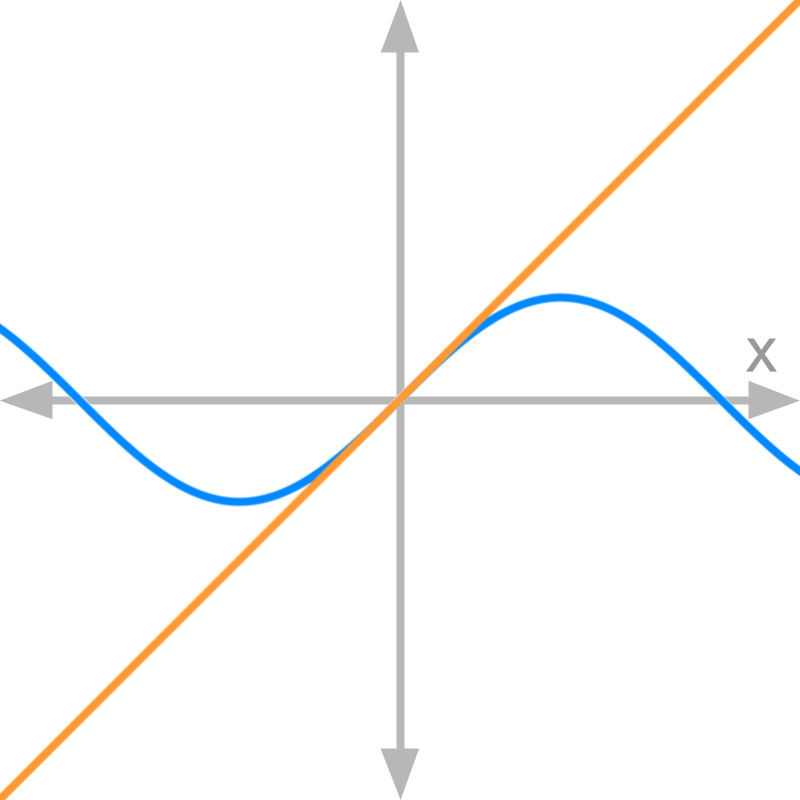Consider the graphs of numerator (in blue) and denominator (in orange) of function $f \left(x\right)$.

The value of ${\lim}_{x \to 0} f \left(x\right) = 1$. This is because, the slope of the numerator equals that of the denominator at the given point.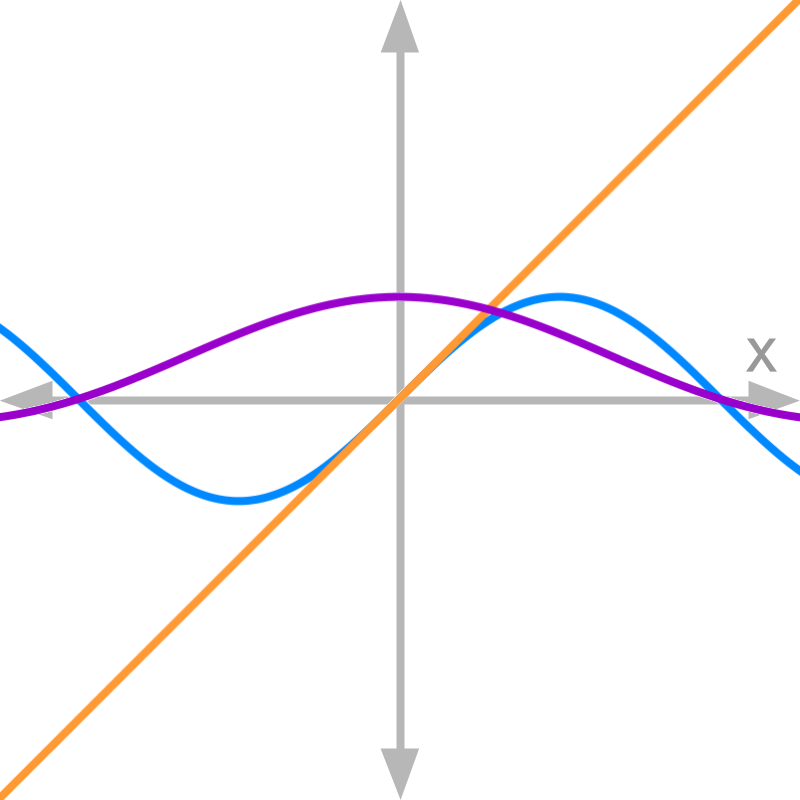Consider the graphs of numerator (in blue) and denominator (in orange) of function $f \left(x\right)$ (in purple).

The function given is $\textcolor{p u r p \le}{f \left(x\right)} = \frac{\textcolor{\mathrm{de} e p s k y b l u e}{\sin x}}{\textcolor{c \mathmr{and} a l}{x}}$. At $x = 0$ the slope of the numerator is $1$ and slope of the denominator is $1$.

Proof for limit of this function is explained later.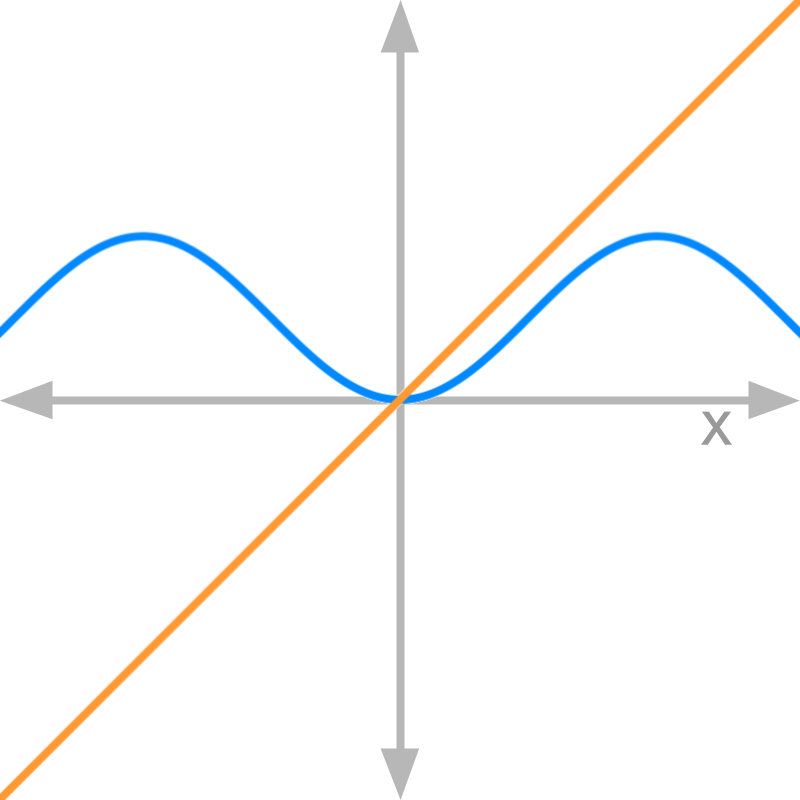Consider the graphs of numerator (in blue) and denominator (in orange) of function $f \left(x\right)$.

The value of ${\lim}_{x \to 0} f \left(x\right) = 0$. This is because, the slope of the numerator is $0$ and that of the denominator is $1$.

slope = 0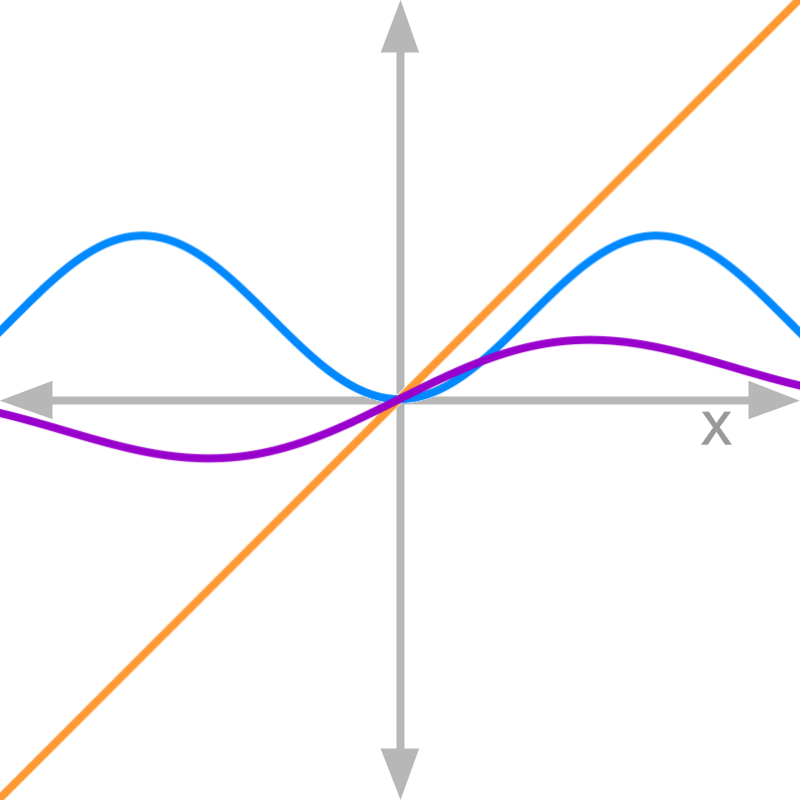Consider the graphs of numerator (in blue) and denominator (in orange) of function $f \left(x\right)$ (in purple).

The function given is $f \left(x\right) = \frac{\textcolor{\mathrm{de} e p s k y b l u e}{1 - \cos x}}{\textcolor{c \mathmr{and} a l}{x}}$. The slope of the numerator is $0$ and the slope of the denominator is $1$.

Proof for limit of this function is explained later.

don't analyse with limit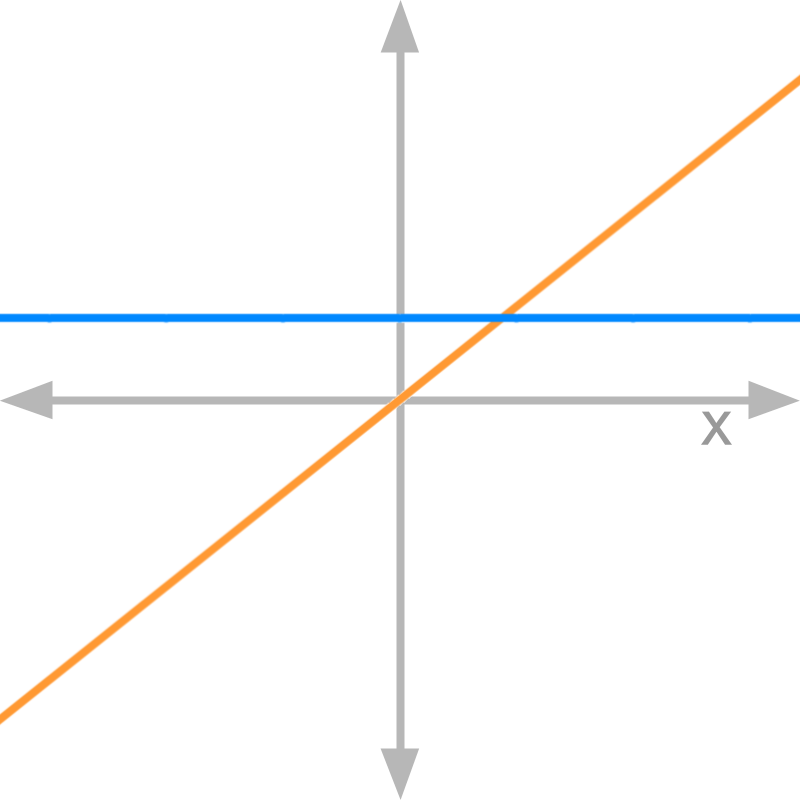Consider the graphs of numerator (in blue) and denominator (in orange) of function $f \left(x\right)$.

The value of ${\lim}_{x \to 0 +} f \left(x\right) = \infty$. This function is not a candidate to analyze numerator and denominator, as the function does not evaluate to $\frac{0}{0}$ form.

don't limit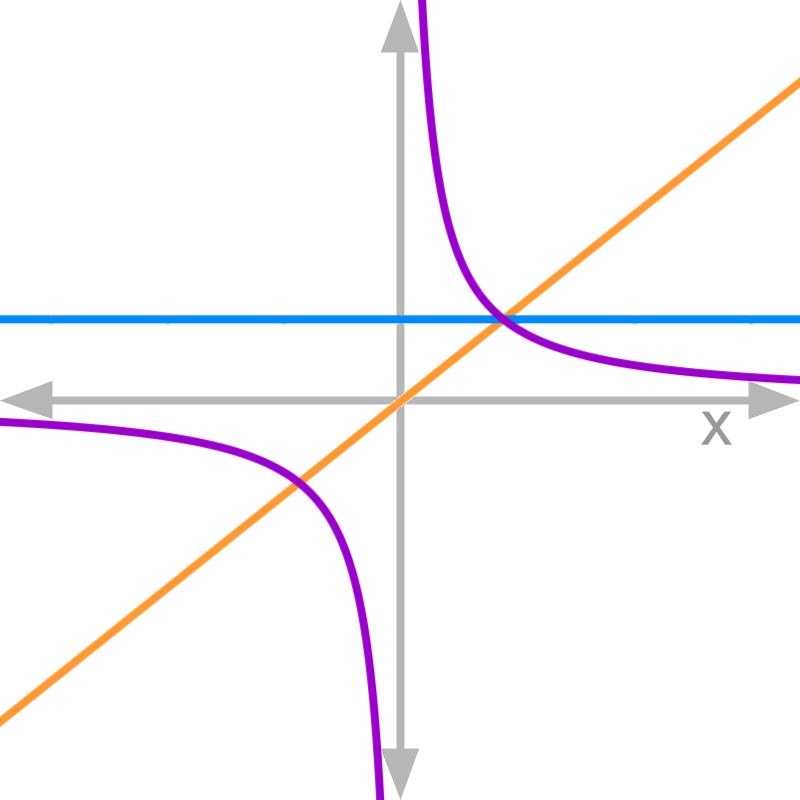Consider the graphs of numerator (in blue) and denominator (in orange) of function $f \left(x\right)$ (in purple).

The function given is $f \left(x\right) = \frac{\textcolor{\mathrm{de} e p s k y b l u e}{1}}{\textcolor{c \mathmr{and} a l}{x}}$.
This function does not satisfy the pre-condition that numerator and denominator has to evaluate to $0$. So the analysis by comparing slopes is not applicable.

exampleConsider the graphs of numerator (in blue) and denominator (in orange) of function $f \left(x\right)$.

The answer is '$0$'. The slope of numerator is $0$ and that of the denominator is $1$.

summary

Slopes of the numerator and denominator at the point $x = a$ decide the limits of $f \left(x\right)$ at $x = a$.
»  eg: $f \left(x\right) = \frac{\textcolor{\mathrm{de} e p s k y b l u e}{2 x - 4}}{\textcolor{c \mathmr{and} a l}{x - 2}}$→  slope of numerator at $x = 2$ is $2$

→  slope of denominator at $x = 2$ is $1$

→  Both LHL and RHL limits $= \frac{2}{1} = 2$

Outline